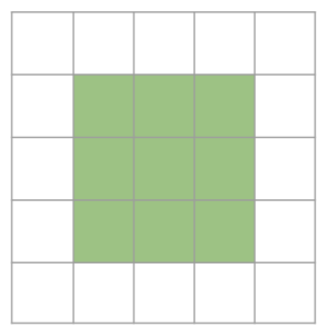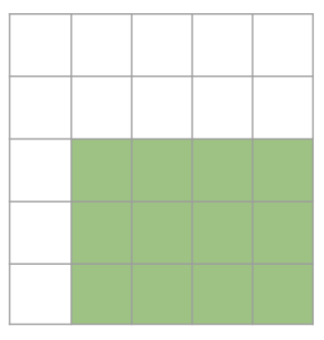시간 제한 메모리 제한 제출 정답 맞은 사람 정답 비율
1 초 512 MB 0 0 0 0.000%

## 문제

I can be a square, or a rectangle. Am I a square, or a rectangle?

Consider a grid of N by N squares. Suppose there is either a square or a rectangle covering some grid squares, and each grid square is either completely inside or outside the shape, i.e. the boundary of the shape follows the grid lines. Two examples are shown below:Figure 1: I am a square. Figure 2: I am a rectangle.

It is also guaranteed that the shape covers at least 4% of the total area of the grid. Your task is to determine if the shape is a square, or a rectangle. To do that, you are allowed to ask at most Q queries, each query allows you to find out if a certain grid square is inside the shape. The grid squares are identified by coordinates (x, y) where 1 ≤ x, y ≤ N.

## 구현

This is an interactive task. Do not read from standard input or write to standard output.

You are to implement the following function:

• bool am_i_square(int N, int Q)

The am_i_square function will be called at most T times. Each call to this function represents a separate instance of the problem, i.e. the shape may be different and Q queries are allowed in each instance of the problem.

Within the am_i_square function, you are allowed to call the following grader functions to complete the task:

• bool inside_shape(int X, int Y)

The inside_shape function will return true if the grid square (X, Y ) is inside the shape, or false otherwise. If your program calls this function more than Q times or with invalid parameters, the program will terminate immediately and you will be given a Wrong Answer verdict.

• N = 100
• 1 ≤ T ≤ 1000

• Q = 104

• Q = 100

## 서브태스크 3 (18점)

• Q = 40
• shape will cover at least 25 % of the grid area.

• Q = 50

## 예시

Consider the grid found in Figure 1. Suppose Q = 25. Your function will be called with the following parameters:

• am_i_square(5, 25)

A possible interaction could be as follows:

• inside_shape(3, 3) = true
The inside_shape function is asked if (3, 3) is inside the shape. As the grid square is inside the shape (highlighted in green as shown in Figure 1), the function returns true.
• inside_shape(5, 4) = false
The inside_shape function is asked if (5, 4) is inside the shape. As the grid square on the fifth row and fourth column is outside the shape, the function returns false.
• inside_shape(1, 1) = false
The inside_shape function is asked if (1, 1) is inside the shape. As the grid square on the top-left corner is outside the shape, the function returns false.
• inside_shape(2, 4) = true
The inside_shape function is asked if (2, 4) is inside the shape. As the grid square is within the shape, the function returns true.

At this point, it decides that it has enough information to conclude that the shape is a square. As such, it will return true. As the shape is indeed a square and the program has used less than 25 queries, it would be deemed as correct for this testcase.

## 제출할 수 있는 언어

C++14, C++17, C++2a, C++14 (Clang), C++17 (Clang), C++2a (Clang)

## 채점

• 예제는 채점하지 않는다.